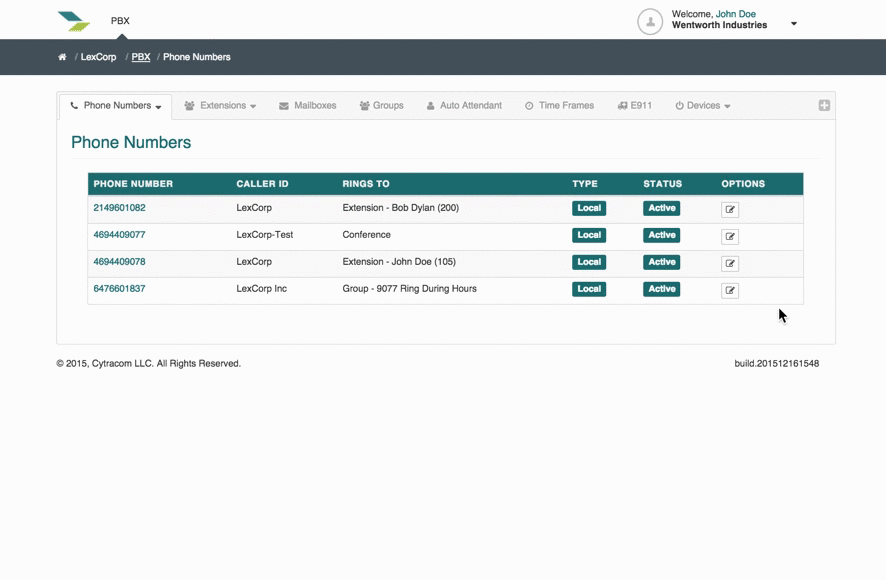# Advanced Device Configuration

Note: Use caution when changing P Values, because if not done correctly, the phone may not register.

Advanced configurations for Grandstream phones can be made for each device individually or on a Device Template. Changing these P Values can override the settings that are already present on the device. Find the Advanced button in the first section of the Device Template or individual Device tab of the PBX.In Chrome, open the web interface of a given phone using the IP address of the device. Right click and choose inspect on any field to see the P Value and its setting.

These settings can be put into a Device Template for all of the same model of phone, and then applied to those phones. It can also be added to devices on an individual basis.

See list below for common Grandstream P Values

----------------------------------------------------------------------------------------------------------------------------

Default Admin Password

 P2=ccomadmin "ccomadmin" is the default password we use if the firmware requires a change

Upgrade Firmware

 P238=0P212=1P192=fw.cytracom.com Sets the phone to update, use the HTTP protocol and pull the latest firmware release supported by Cytracom (For HTTPS use P212=2)

Time, Display, and Weather

 P143=0P144=0P64=P30=pool.ntp.org For the value section enter the desired timezone using the following syntax: PST8PDT|MST7MDT|CST6CDT|EST5EDT P2918=<1 On, 0 Off>P2916=4P2921=P2916=3P2917= Screen Saver On or Off   Wallpaper: Color value is in Hex code: #000000   Wallpaper: HTTP server address link to a compatible JPG P8338=0P1402=1P1405=0P1377= Weather service uses zipcode for determining the location.

Dial Plan

 P290={x+|x+*|*x+|*66x+*x+} Latest Dial Plan supports Dynamic Caller ID

Server Registration

 P130=0P2329=1P47=register.cytracom.netP40=5060 UDP Normal Port Configuration P130=0P2329=1P47=register.cytracom.net:5062P40=5062 UDP Secondary Port Configuration P130=2P2329=0P47=register.cytracom.netP40=5061 TLS Encryption using TCP P130=1P2329=0P47=register.cytracom.netP40=5060 TCP using normal port configuration

Ring Tone

 P1488= P1489= Value is usually an extension format such as xxx to perform an internal vs external ringtone, you can even differentiate between multiple extension zones such as 1xx, 2xx, and 3xx 1 - High pitch classic with number announce2 - Four tone Grandstream Classic3 - Medium Pitch

Multi-Cast Paging

 P1568=0P1569=224.0.0.0:987P1570=Main Listen P323=13P301=0P302=MainP303=224.0.0.0:987 MPK 1 P1367=23P1368=0P1469=MainP1470=224.0.0.0:987 Line 3

Disable Incoming Call Waiting Tone

 P186=1 -

Disable Call Waiting

 P26063=2 -

Disable SIP URI Schema being displayed

 P2380=0P2324=2P26025=2 In some scenarios the SIP information is displayed depending on the network. This will disable that or prevent the network from changing the SIP information.

OnHook Dial Barge

 P8397=0 -

Rogue Calls

 P40=5078P2306=1P258=0 -

Disable Active MPK

 P6764=1 -

Log In/Out Softkeys

 P2987=10P2988=0P2989=Log InP2990=*01 P2991=10P2992=0P2993=Log OutP2994=*00 Adds a Log In key to the Softkeys on the screen of the phone. Agent # is the agent number that they are in Call Center.     Adds a Log out key to the Softkeys on the screen of the phone.

----------------------------------------------------------------------------------------------------------------------------

For a complete list of Grandstream P-Values, see the attachment.

Was this article helpful?
0 out of 1 found this helpful
Have more questions? Submit a request